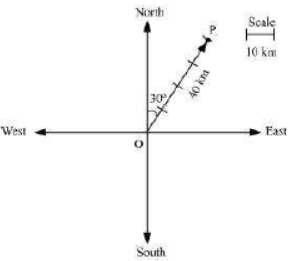# NCERT Class 12 Maths Solutions Vector Algebra

## Vector Algebra Class 12 NCERT Solutions

Class 12 Mathematics students should refer to the following NCERT questions with answers for Vector Algebra in standard 12. These NCERT Solutions with answers for Grade 12 Mathematics will come in exams and help you to score good marks

### Vector Algebra NCERT Solutions Class 12

MATHS

Represent graphically a displacement of 40 km, 30° east of north.Here, vector represents the displacement of 40 km, 30° East of North.

Question 2:

Classify the following measures as scalars and vectors.

(i) 10 kg (ii) 2 metres north-west (iii) 40°

(iv) 40 watt (v) 10–19 coulomb (vi) 20 m/s2

(i) 10 kg is a scalar quantity because it involves only magnitude.

(ii) 2 meters north-west is a vector quantity as it involves both magnitude and direction.

(iii) 40° is a scalar quantity as it involves only magnitude.

(iv) 40 watts is a scalar quantity as it involves only magnitude.

(v) 10–19 coulomb is a scalar quantity as it involves only magnitude.

(vi) 20 m/s2 is a vector quantity as it involves magnitude as well as direction.

Question 3:

Classify the following as scalar and vector quantities.(i) time period (ii) distance (iii) force

## Books recommended by teachers

 NCERT Class 12 Maths Solutions Relations and Functions
 NCERT Class 12 Maths Solutions Inverse Trigonometric Functions
 NCERT Class 12 Maths Solutions Matrices
 NCERT Class 12 Maths Solutions Determinants
 NCERT Class 12 Maths Solutions Continuity and Differentiability
 NCERT Class 12 Maths Solutions Application of Derivatives
 NCERT Class 12 Maths Solutions Integrals
 NCERT Class 12 Maths Solutions Application of Integrals
 NCERT Class 12 Maths Solutions Differential Equations
 NCERT Class 12 Maths Solutions Vector Algebra
 NCERT Class 12 Maths Solutions Three Dimensional Geometry
 NCERT Class 12 Maths Solutions Linear Programming
 NCERT Class 12 Maths Solutions Probability